# Evaluating Reflective Measurement Model. Step 4: Discriminant Validity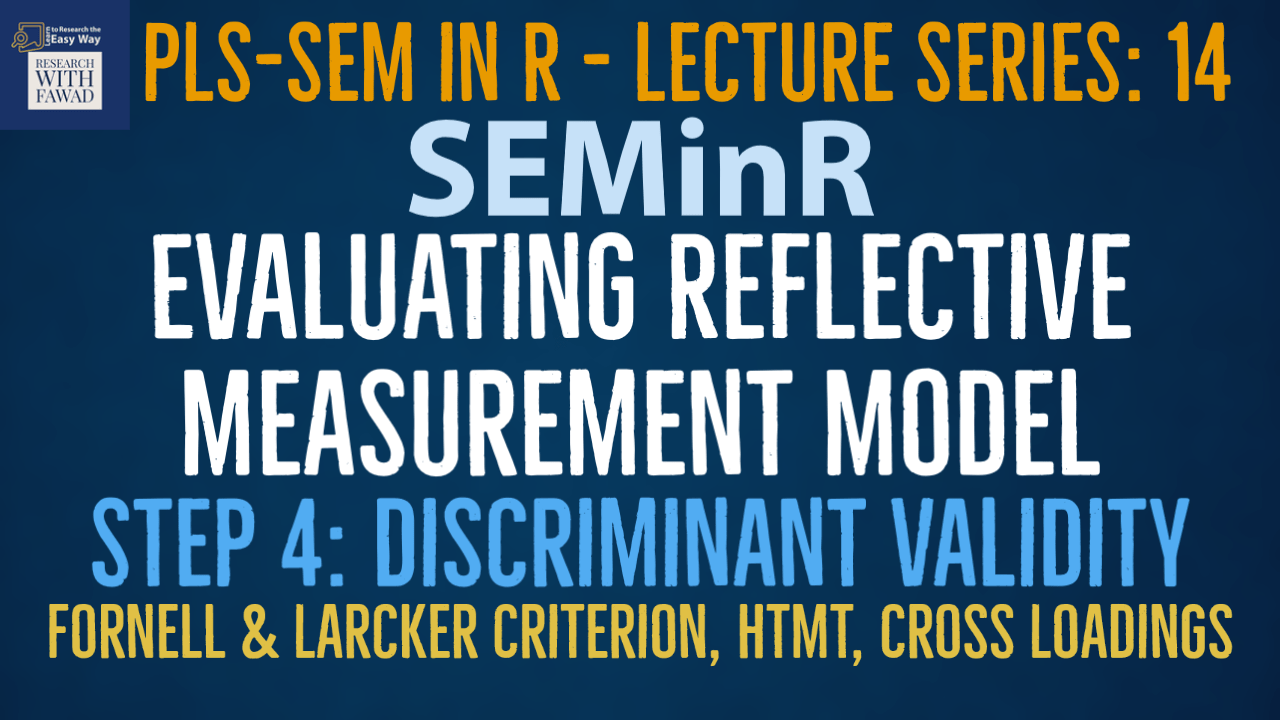### SEMinR Lecture Series

This series of lectures on SEMinR Package will focus on evaluating reflective measurement model.

## Evaluating Reflective Measurement Model

• In the last session the focus was on assessing indicator reliability.
• This lecture will focus on Step 2: Internal Consistency and Step 3: Convergent Validity
• The figure illustrates the reflective measurement model evaluation process.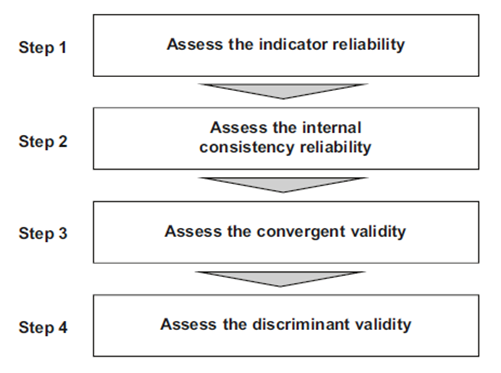## Discriminant Validity

• The fourth step is to assess discriminant validity. This metric measures the extent to which a construct is empirically distinct from other constructs in the structural model.
• Fornell and Larcker (1981) proposed the traditional metric and suggested that each construct’s AVE (squared variance within) should be compared to the squared inter-construct correlation (as a measure of shared variance between constructs) of that same construct and all other reflectively measured constructs in the structural model – the shared variance between all model constructs should not be larger than their AVEs.
• Recent research indicates, however, that this metric is not suitable for discriminant validity assessment. For example, Henseler, Ringle, and Sarstedt (2015) show that the Fornell–Larcker criterion (i.e., FL in SEMinR) does not perform well, particularly when the indicator loadings on a construct differ only slightly (e.g., all the indicator loadings are between 0.65 and 0.85).
• Hence, in empirical applications, the Fornell–Larcker criterion often fails to reliably identify discriminant validity problems (Radomir & Moisescu, 2019) and should therefore be avoided. Nonetheless, it is included in the tutorials, as many researchers are familiar with it.
• An alternative is the heterotraitmonotrait ratio (HTMT) of correlations (Henseler et al., 2015) to assess discriminant validity.
• Figure illustrates this concept. The arrows connecting indicators of different constructs represent the heterotrait–heteromethod correlations, which should be as small as possible.
• On the contrary, the monotrait–heteromethod correlations – represented by the dashed arrows – represent the correlations among indicators measuring the same concept, which should be as high as possible.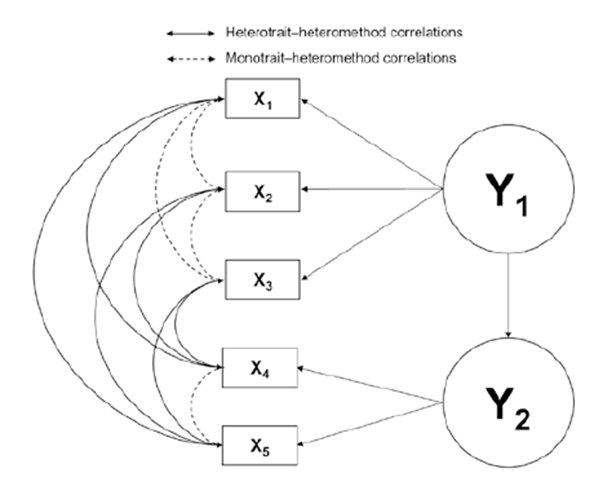## Assessing Discriminant Validity

• Discriminant validity problems are present when HTMT values are high.
• Henseler et al. (2015) propose a threshold value of 0.90 for structural models with constructs that are conceptually very similar, such as cognitive satisfaction, affective satisfaction, and loyalty.
• In such a setting, an HTMT value above 0.90 would suggest that discriminant validity is not present.
• But when constructs are conceptually more distinct, a lower, more conservative, threshold value is suggested, such as 0.85 (Henseler et al., 2015).
• SEMinR offers several approaches to assess discriminant validity. According to the Fornell–Larcker criterion (Fornell & Larcker, 1981), the square root of the AVE of each construct should be higher than the construct’s highest correlation with any other construct in the model..
• These results can be checked through the summary_simple object and validity element for the fl_criteria:
• The primary criterion for discriminant validity assessment is the HTMT criterion, which can be accessed by inspecting the summary_simple() object and validity element for the \$htmt.
``````#To Retreive Table of the FL criteria
summary_simple\$validity\$fl_criteria
#To Retreive Table for HTMT criterion
summary_simple\$validity\$htmt``````
• R will provide the results as shown in the figure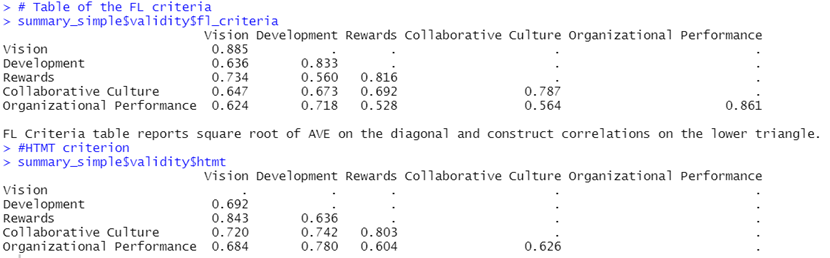## Bootstrapping HTMT Results

• In addition to examining the HTMT values, researchers should test whether the HTMT values are significantly different from 1 or a lower threshold, such as 0.9 or even 0.85.
• This analysis requires computing bootstrap confidence intervals obtained by running the bootstrapping procedure. To do so, use the bootstrap_model() function and assign the output to an object, such as boot_model.
• Then, run the summary() function on the boot_model object and assign it to another object, such as summary_boot. In doing so, we need to set the significance level from 0.05 (default setting) to 0.10 using the alpha argument. In this way, we obtain 90% two-sided bootstrap confidence intervals for the HTMT values, which is equivalent to running a one-tailed test at 5%.
``````# Bootstrap the model for HTMT on the PLS Estimated Model
boot_model <- bootstrap_model(seminr_model = simple_model, nboot = 1000)
Summary_boot <- summary(boot_model, alpha = 0.10)``````
• Bootstrapping should take a few seconds, since it is a processing-intensive operation. As the bootstrap computation is being performed, a red STOP indicator should show in the top-right corner of the console. This indicator will automatically disappear when computation is complete, and the console will display “SEMinR Model successfully bootstrapped.”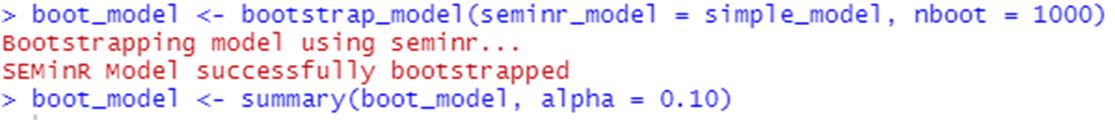• Extract the bootstrapping confidence intervals of the HTMT by inspecting the \$bootstrapped_HTMT of the summary_boot variable:
• Researchers should always use 10,000 bootstrap samples (Streukens & Leroi-Werelds, 2016).
``````# Extract the bootstrapped HTMT
summary_boot\$bootstrapped_HTMT``````
• The output in Fig. displays the original ratio estimates (column: Original Est.), bootstrapped mean ratio estimates (column: Bootstrap Mean), bootstrap standard deviation (column: Bootstrap SD), bootstrap t- statistic (column: T Stat.), and 90% confidence interval (columns: 5% CI and 95% CI, respectively).
• The bootstrapping procedure allows for constructing confidence intervals for the HTMT, In order to test the null hypothesis (H0: HTMT ≥ 1) against the alternative hypothesis (H1: HTMT < 1).
• A confidence interval containing the value one (i.e., H0 holds) indicates a lack of discriminant validity.
• Conversely, if the value one falls outside the interval’s range, this suggests that the two constructs are empirically distinct.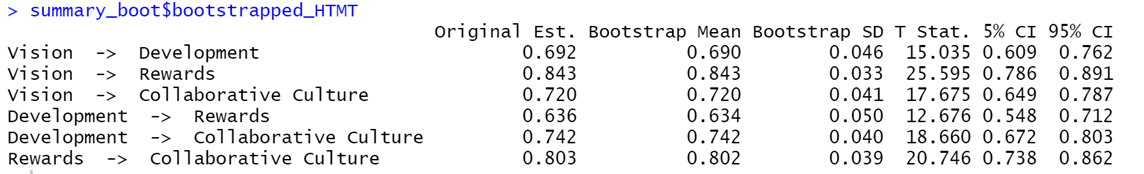``````#Cross Loadings for Assessment of Discriminant Validity

## Measurement Model Summary

• Table summarizes all the metrics that need to be applied when assessing reflective measurement models.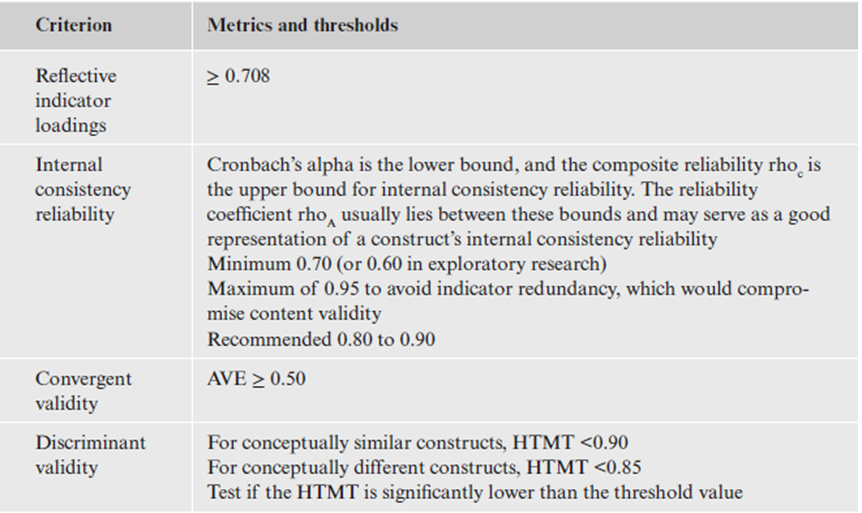## Complete Code

``````library(seminr)
# Create measurement model
simple_mm <- constructs(
composite("Vision", multi_items("VIS", 1:4)),
composite("Development", multi_items("DEV", 1:7)),
composite("Rewards", multi_items("RW",1:4)),
composite("Collaborative Culture", multi_items("CC", 1:6)),
composite("Organizational Performance", multi_items("OP", 1:5)))
# Create structural model
simple_sm <- relationships(
paths(from = c("Vision","Development","Rewards"), to = "Collaborative Culture"),
paths(from = "Collaborative Culture", to = "Organizational Performance"))
# Estimate the model
simple_model <- estimate_pls(data = datas,
measurement_model = simple_mm,
structural_model = simple_sm)
# Summarize the model results
summary_simple <- summary(simple_model)
# Iterations to converge
summary_simple\$iterations
#To Display all the contents in the summary_simple Object
summary_simple
# Inspect the indicator reliability
#Inspect the composite reliability
summary_simple\$reliability
#Plot the reliabilities of constructs
plot(summary_simple\$reliability)
# Table of the FL criteria
summary_simple\$validity\$fl_criteria
#HTMT criterion
summary_simple\$validity\$htmt
# Bootstrap the model for HTMT on the PLS Estimated Model
boot_model <- bootstrap_model(seminr_model = simple_model, nboot = 1000)
summary_boot <- summary(boot_model, alpha = 0.10)
#Generate complete Summary
summary_boot
# Extract the bootstrapped HTMT
summary_boot\$bootstrapped_HTMT